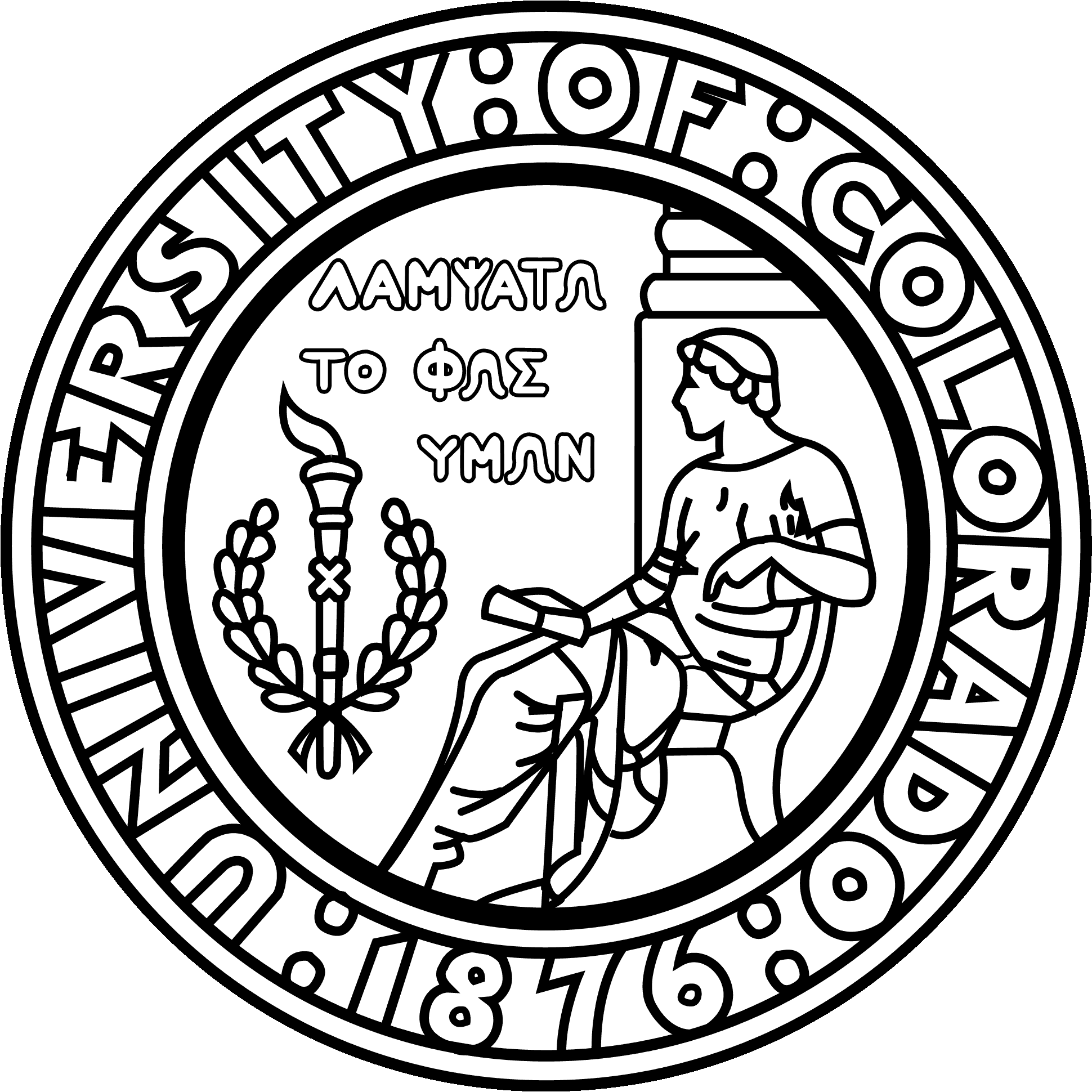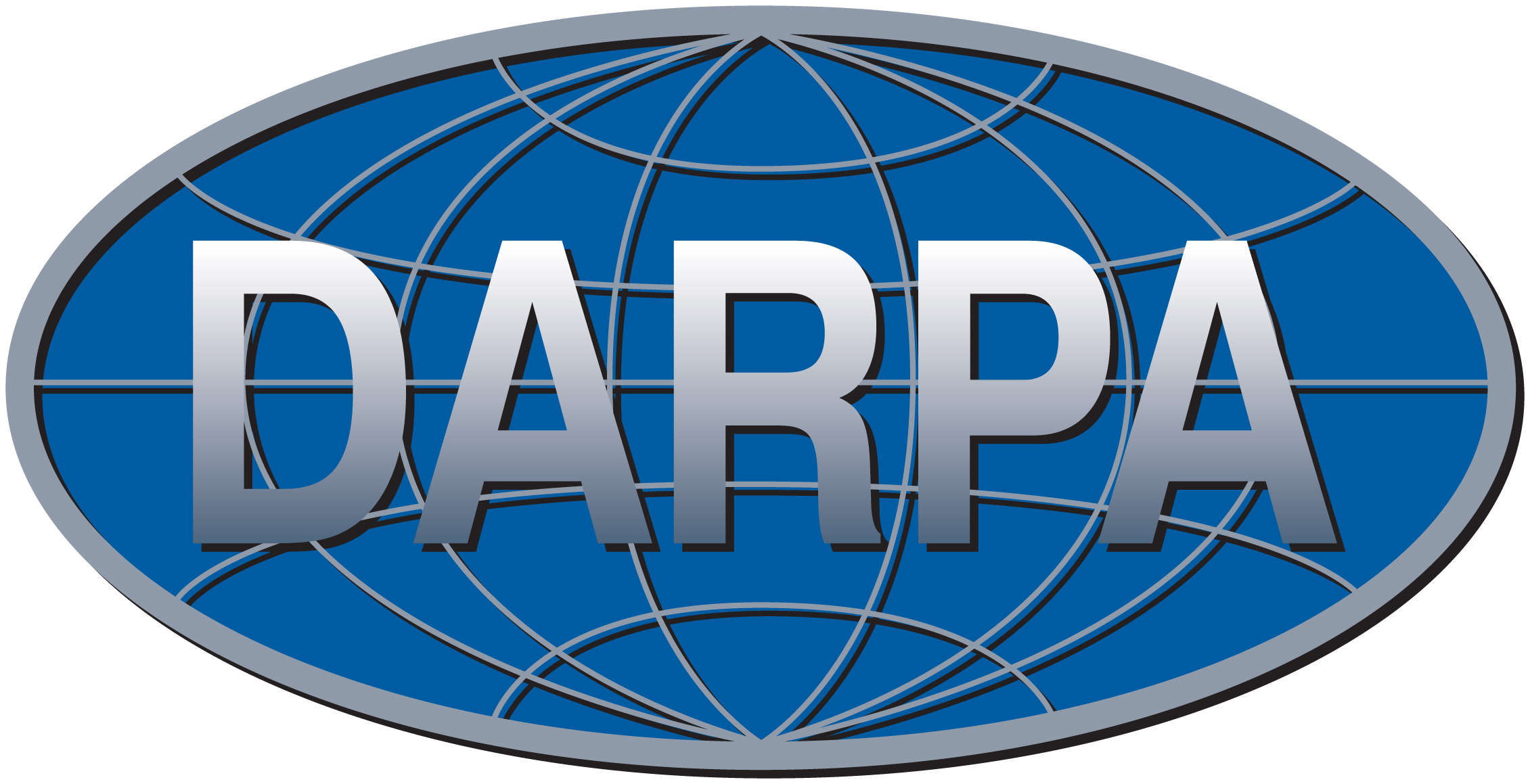CBMS/NSF Conference on Fast Direct Solvers, June 2014

Dartmouth College, June 23 - 27, 2014

 Home Lectures Codes Bibliography Support

 FMM RSVD ID BIEs FDS for sparse FDS for IE Poincaré-Steklov

The Fast Multipole Method

Early descriptions of the FMM include a 1985 paper by Vladimir Rokhlin, and the seminal 1987 JCP paper by Leslie Greengard and VR.

The description of the FMM in Lecture 2 follows this encyclopedia entry.

Detailed descriptions of the FMM are given in, e.g, the dissertation of Leslie Greengard, a short course on FMMs by Rick Beatson and Leslie Greengard, a tutorial by Liu and Nishimura, and a variety of books, including this by Chew, Jin, Michielssen, and Son, and this by Yijun Liu.

Adaptivity is described in a 1988 SISC paper by Carrier, Greengard, and Rokhlin.

An essential improvement to the FMM that allows high speed for Laplace problems in 3D is described here.

The profoundly remarkable fact that FMMs can be constructed for high-frequency Helmholtz problems (where, recall, the rank considerations used for Laplace no longer work) was discovered by Rokhlin and is published here and here. For a more comprehensive description of these algorithms, see this.

An FMM based on the interpolative decomposition (ID) is described here.

A general collection of references on the FMM is to be found at fastmultipole.org.

Randomized methods for low-rank approximation

This survey provides an accessible introduction. It is 72 pages long, but the introduction (Section 1) can be read on its own and provides a brief introduction.

Another, briefer, introduction is given in this 2011 PNAS paper.

The original paper we published on these methods is this 2006 tech report. The report was (after two rejections...) eventually published here in ACHA.

For a full description of the intellectual pedigree of these methods, please see the survey mentioned earlier, and also this survey by Michael Mahoney.

The interpolative decomposition (ID)

For a technical paper, see this.

Randomized techniques for building the ID are described in Section 5.2 of this survey.

A seminal 1996 SISC paper by Gu and Eisenstat provides much of the mathematical underpinnings. Earlier work on the related Rank-Revealing QR (RRQR) factorization includes a 1987 paper by Tony Chan.

Applications to functions of a continuum variable are described here.

Boundary integral equations and Nyström discretization

Standard references include "Linear Integral Equations" by Rainer Kress (oddly, the full pdf seems to be available here!), and "The Numerical Solution of Integral Equations of the Second Kind" by Kendall Atkinson. For a more mathematical treatment, see "Strongly elliptic systems and boundary integral equations" by William McLean.

An introduction intended to be highly accessible can be found here. This intro is still a little rough, but should provide a quick access point.

Methods for high-order discretization of BIEs in 2D with singular kernels are described here. (Local copy.)

A brief introduction to methods for handling corners can be found here. (Local copy.) Original work in this area by James Bremer and Johan Helsing.

Direct solvers for sparse matrices from FEM/FD discretization

A slightly outdated, but (I think!) accessible description of the ideas can be found in this 2009 paper. (Local copy.) This is pursued further in the dissertation of Adrianna Gillman. A journal publication on this work is here.

Work on so called H-LU methods which use H-matrix algebra to accelerate the nested dissection method by Lars Grasedyck, Sabine Le Borne, and Ronald Kriemann can be found in this 2007 paper.

A 2009 SIMAX paper by J. Xia, S. Chandrasekaran, M. Gu, and X.S. Li introduced many ideas, and was later followed up by a long sequence of papers by Jianlin Xia and co-workers, which can be found on his home page.

Related work by Philip Schmitz and Lexing Ying is here, and by Ken Ho and Lexing Ying here.

For a rough introduction to matrix algebra for rank-structured matrices, see this rough extract from the monograph manuscript.

Original references for H-matrix algebra include a 1999 paper in Computing by Wolfgang Hackbusch. The H2-matrix arithmetic was introduced to reduce complexity from O(N (log N)k) to O(N) and is described in this 2000 paper by W. Hackbusch, B. Khoromskij, and S. A. Sauter. A 2008 book by Mario Bebendorf provides a full reference to H-matrix techniques, and a 2010 book by Steffen Börm describes H2-matrix methods.

Resources on H-matrices, including codes and an extensive bibliography, can be found at hlib.org.

So called hierarchically off-diagonal low-rank (HODLR) matrices are used in this 2014 manuscript by A. Aminfar, S. Ambikasaran, and E. Darve. This manuscript also contains a very informative literature survey.

Direct solvers for integral equations

The original paper describing "hierarchical skeletonization" is this 2005 paper, which in turn builds off of a single-level scheme described here. This survey article describes essentially the same method, but now applied in 3D (local copy). A cleaner description of the same algorithm, which makes the connection to HBS matrix algebra explicit, can be found in this 2012 paper (local copy). A variation of the same scheme, which uses a general purpose sparse structured solver (UMFPACK) by Greengard and Ho can be found here. A variation that appears to attain O(N) complexity by Ho and Ying can be found here.

An extension to 3D based on hierarchical merging of scattering matrices can be found here (to appear in BIT).

Acceleration to optimal O(N) complexity for 2D volume integral equations (e.g. Lippman-Schwinger) is described in this 2014 ACHA paper. Optimal complexity is attained by using HBS matrix algebra for the scattering matrices.

Direct solvers for integral equations based on H-matrix methods are described in the following two books: Efficient Numerical Methods for Non-local Operators H2-Matrix Compression, Algorithms and Analysis, by Steffen Borm, and Hierarchical Matrices, by Mario Bebendorf.

Exciting very recent work in Eric Michielssen's group seems to show that so called "butterfly" algorithms (see, e.g., this 2010 ACHA paper by M. O'Neil, F. Woolfe, and V. Rokhlin) can be used to build linear complexity algorithms for high frequency scattering problems.

The Hierarchical Poincaré-Steklov scheme

Original paper describing the method is here (local copy).

Acceleration to O(N) complexity is described here (to appear in SISC).

Coupling with a BIE for the external domain to solve free space scattering here (local copy).

Miscellaneous references (unsorted):

Research support by:P.G. Martinsson, June 2014